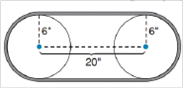Chapter 8.4, Problem 40EElementary Geometry For College St...

7th Edition
Alexander + 2 others
ISBN: 9781337614085

Solutions

Chapter
SectionElementary Geometry For College St...

7th Edition
Alexander + 2 others
ISBN: 9781337614085
Textbook Problem

In exercises 34-45, use your calculator value of π unless otherwise stated. Round answers to two decimal places.In a two pulley system, the center of the pulleys is 20 in apart. If the radius of the each pulley measures 6 in., how long is the belt used in the pulley system.To determine

To Find:

The length of the belt used in pulley system.

Explanation

The distance between the center of the pulleys is 20 in.

The distance of the belt on both sides is 20+20=40

A total distance of the belt for pulleys on both sides is 40 in.

The radius of the pulley is 6 in.

The belt is covered the pulleys semicircle on both sides.

Therefore, the belt forms a circle over the pulleys.

The circumference of the circular belt is C=2πr

Still sussing out bartleby?

Check out a sample textbook solution.

See a sample solution

The Solution to Your Study Problems

Bartleby provides explanations to thousands of textbook problems written by our experts, many with advanced degrees!

Get Started

In Exercises 4756, solve the given equation for the indicated variable. 4=2x

Finite Mathematics and Applied Calculus (MindTap Course List)

Evaluate the integrals in Problems 7-18. 10.

Mathematical Applications for the Management, Life, and Social Sciences

Evaluate the integral. 16. 02/2x21x2dx

Single Variable Calculus: Early Transcendentals